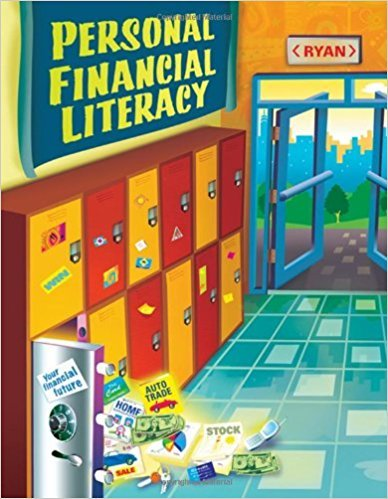×
Get Full Access to Personal Financial Literacy - 1 Edition - Chapter 2-2 - Problem 1
Get Full Access to Personal Financial Literacy - 1 Edition - Chapter 2-2 - Problem 1

×

# How is unearned income different from earned incomeISBN: 9780538444521 464

## Solution for problem 1 Chapter 2-2

Personal Financial Literacy | 1st Edition

• Textbook Solutions
• 2901 Step-by-step solutions solved by professors and subject experts
• Get 24/7 help from StudySoup virtual teaching assistantsPersonal Financial Literacy | 1st Edition

4 5 1 393 Reviews
14
5
Problem 1

How is unearned income different from earned income?

Step-by-Step Solution:
Step 1 of 3

MAT210 BriefCalculus ArizonaStateUniversity Lectureby:JayAbramson 11.2MarginalAnalysis Profit=RevenueCost Marginal=Derivative P(x)=R(x)-C(x) P’(x)=R’(x)-C’(x) P(x)=(price)x-C(x) C(10)=2000 Costtoproduce10itemsus$2000 C’(10)=5 11thitemis$5 C(11)-C(10)=5.50 Exactcostof11thitemis$5.50 Example1 C(x)=0.025x +8x+10 Costtoproducexitems Eachitemsellsfor 10-0.01x (price) a) Findthemarginalcostatx=10 C’(x)=0.05x+8 C’(10)=0.05(10)+8=$8.50 b) Findtheexactcostofthe11t

Step 2 of 3

Step 3 of 3

##### ISBN: 9780538444521

Unlock Textbook Solution